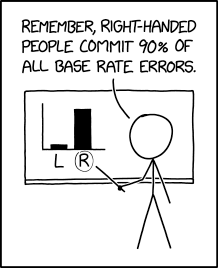The coronavirus pandemic has changed quite a lot. One of the lesser important things is that people in typical math problems are not as far-fetched anymore as they used to be. And no, I’m not talking about people buying 1000 eggs or 200 rolls of toilet paper. No, I’m talking about math exercises concerned with Bayes’ Theorem. This articles answers: What is Bayes’ Theorem, and why is it relevant?

In this article, I will not employ mathematical rigor. This should make the article much more accessible. If you want a more in-depth explanation, head over to Bayes’ Theorem on Wikipedia, or even better any math book on the topic.

## Bayes’ Theorem

Let’s get started. What is Bayes’ Theorem? The gist is perfectly captured the XKCD comic titled Base Rate:Randall lets Cueball commit a base rate error in an ironic way. A less ironic version of the comic could read, “Right-handed people cause 90% of all car accidents”. This statement might be accurate, but nobody would give it any credit. It neglects the fact that the group of right-handed people constitute the majority of the population. There is presumably no correlation (not even speaking of causation) between a person’s handedness and the person’s tendency to cause car accidents.

The ridiculousness of statements like Right-handed people cause 90% of all car accidents should be obvious. The urge is to correct this for the prevalence of right- and left-handedness in the entire population. But, what is the correct way to account for that fact. Let me introduce: conditional probabilities and Bayes’ Theorem.

Tossing mathematical rigor, most people have a feeling for what the Probability of event $$A$$ means (although “probably” flawed). Let’s write this as $$P(A)$$. Examples would be

• $$P(\text{a person is right-handed})$$ or
• $$P(\text{a person causes a car accident})$$.

Conditional probabilities capture the probability of an event $$B$$ if we assume another event already happened. This sounds like we are restricted to events on a timeline, but we can understand events in a more general sense of properties with no causal connection. Formally, we write $$P(B\vert A)$$, and say probability of $$B$$ given $$A$$. We can use our two examples from above to build conditional probabilities:

• $$P(\text{a person is right-handed} \vert \text{the same person causes a car accident})$$ or
• $$P(\text{a person causes a car accident} \vert \text{the same person is right handed})$$.

Take a moment to understand what these two probabilities mean. Although they seem similar, the probabilities are very much different. To make the difference clearer, consider the two conditional $$P(\text{a person is pregnant}\vert \text{the same person is a woman})$$ and $$P(\text{a person is a woman}\vert \text{the same person is pregnant})$$. I don’t know the number in the first case, but the answer to the second probability is 1. If a person is pregnant, it follows that the person is a woman.

Now that we have conditional probabilities, we can introduce Bayes’ Theorem. Bayes’ Theorem relates two conditional probabilities where the arguments are swapped, like in the above cases:

$P(A\vert B) = \frac{P(A) P(B\vert A)}{P(B)}$

I will not try to derive or motivate Bayes’ Theorem here. Many smart people have done this already.

## To vaccinate or not to vaccinate

Why is Bayes’ Theorem suddenly relevant? Well, it was always relevant. Many textbook examples for Bayes’ Theorem discuss the effectiveness of a medical test for a rare disease and what it actually means when said test is positive.

In this article, I want to work out the probabilities that a person requires treatment in an intensive care unit (ICU) depending (ringing bell, i.e., conditional probabilities) on the persons’ coronavirus vaccination status. As a disclaimer, I don’t actually care to use the latest and most precise numbers, or consider all the medial nuances. All I want to show is how to compute this in general. That fact that I plug in numbers in the end is just a bonus.

Formally, we want to compute the two probabilities

• $$P(\text{ICU}\vert \text{vaccinated})$$ and
• $$P(\text{ICU}\vert \text{not vaccinated})$$.

Media outlets report the number of vaccinated $$n_v$$ and unvaccinated $$n_u$$ people currently receiving treatment for COVID-19 in ICUs. From this, we can estimate

\begin{align*} P(\text{vaccinated} \vert \text{ICU}) &= \frac{n_v}{n_v + n_u}\\ P(\text{not vaccinated} \vert \text{ICU}) &= \frac{n_u}{n_v + n_u} \end{align*}

By now, you should realize that these two probabilities are different from the ones we are looking for, but are exactly the kind of probabilities that we can related with Bayes’ Theorem. To apply the theorem, we need to find out the two unconditional probabilities $$P(\text{vaccinated}) = 1 - P(\text{not vaccinated})$$ and

$P(\text{ICU}) = \frac{n_v + n_u}{n}$

where $$n$$ is the total number of people in the relevant area. Putting everything together yields

\begin{align*} P(\text{ICU}\vert \text{vaccinated}) &= \frac{n_v}{n P(\text{vaccinated})}\\ P(\text{ICU}\vert \text{not vaccinated}) &= \frac{n_u}{n - nP(\text{vaccinated})} \end{align*}

Plugging in numbers for the area where I live, I get the probability to require ICU treatment for COVID-19 between September 13 and October 10, depending on the vaccination status:

\begin{align*} P(\text{ICU}\vert \text{vaccinated}) &= 3.5\cdot 10^{-6}\\ P(\text{ICU}\vert \text{not vaccinated}) &= 2.5\cdot 10^{-5} \end{align*}

So it was more than 7 times more likely to require ICU treatment if a person was not vaccinated.